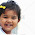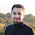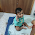VHDL coding tips and tricks: VHDL code for a 4 tap FIR filter

## Saturday, June 25, 2011

### VHDL code for a 4 tap FIR filter

Finite Impulse Response(FIR) filters are one of the two main type of filters available for signal processing. As the name suggests the output of a FIR filter is finite and it settles down to zero after some time. For a basic FAQ on FIR filters see this post by dspguru.
A FIR filter output, 'y' can be defined by the following equation:Here, 'y' is the filter output, 'x' in the input signal and 'b' is the filter coefficients. 'N' is the filter order. The higher the value of N is, the more complex the filter will be.

For writing the code in VHDL I have referred to the paper, VHDL generation of optimized FIR filters. You can say I have coded the exact block diagram available in the paper, "Figure 2".

This is a 4 tap filter. That means the order of the filter is 4 and so it has 4 coefficients. I have defined the input as signed type of 8 bits wide. The output is also of signed type with 16 bits width. The design contains two files. One is the main file with all multiplications and adders defined in it, and another for defining the D flip flop operation.

The main file is given below:

library IEEE;
use IEEE.STD_LOGIC_1164.ALL;
use IEEE.NUMERIC_STD.ALL;

entity fir_4tap is
port(   Clk : in std_logic; --clock signal
Xin : in signed(7 downto 0); --input signal
Yout : out signed(15 downto 0)  --filter output
);
end fir_4tap;

architecture Behavioral of fir_4tap is

component DFF is
port(
Q : out signed(15 downto 0);      --output connected to the adder
Clk :in std_logic;      -- Clock input
D :in  signed(15 downto 0)      -- Data input from the MCM block.
);
end component;

signal H0,H1,H2,H3 : signed(7 downto 0) := (others => '0');
signal MCM0,MCM1,MCM2,MCM3,add_out1,add_out2,add_out3 : signed(15 downto 0) := (others => '0');
signal Q1,Q2,Q3 : signed(15 downto 0) := (others => '0');

begin

--filter coefficient initializations.
--H = [-2 -1 3 4].
H0 <= to_signed(-2,8);
H1 <= to_signed(-1,8);
H2 <= to_signed(3,8);
H3 <= to_signed(4,8);

--Multiple constant multiplications.
MCM3 <= H3*Xin;
MCM2 <= H2*Xin;
MCM1 <= H1*Xin;
MCM0 <= H0*Xin;

--adders
add_out1 <= Q1 + MCM2;
add_out2 <= Q2 + MCM1;
add_out3 <= Q3 + MCM0;

--flipflops(for introducing a delay).
dff1 : DFF port map(Q1,Clk,MCM3);
dff2 : DFF port map(Q2,Clk,add_out1);
dff3 : DFF port map(Q3,Clk,add_out2);

--an output produced at every positive edge of clock cycle.
process(Clk)
begin
if(rising_edge(Clk)) then
Yout <= add_out3;
end if;
end process;

end Behavioral;

VHDL code for the component DFF is given below:

library IEEE;
use IEEE.STD_LOGIC_1164.ALL;
use IEEE.NUMERIC_STD.ALL;

entity DFF is
port(
Q : out signed(15 downto 0);      --output connected to the adder
Clk :in std_logic;      -- Clock input
D :in  signed(15 downto 0)      -- Data input from the MCM block.
);
end DFF;

architecture Behavioral of DFF is

signal qt : signed(15 downto 0) := (others => '0');

begin

Q <= qt;

process(Clk)
begin
if ( rising_edge(Clk) ) then
qt <= D;
end if;
end process;

end Behavioral;

I have written a small test bench code for testing the design. It contains 8 test inputs which are serially applied to the filter module. See below:

LIBRARY ieee;
USE ieee.std_logic_1164.ALL;
USE ieee.numeric_std.ALL;

ENTITY tb IS
END tb;

ARCHITECTURE behavior OF tb IS

signal Clk : std_logic := '0';
signal Xin : signed(7 downto 0) := (others => '0');
signal Yout : signed(15 downto 0) := (others => '0');
constant Clk_period : time := 10 ns;

BEGIN

-- Instantiate the Unit Under Test (UUT)
uut: entity work.fir_4tap PORT MAP (
Clk => Clk,
Xin => Xin,
Yout => Yout
);

-- Clock process definitions
Clk_process :process
begin
Clk <= '0';
wait for Clk_period/2;
Clk <= '1';
wait for Clk_period/2;
end process;

-- Stimulus process
stim_proc: process
begin
wait for Clk_period*2;
Xin <= to_signed(-3,8); wait for clk_period*1;
Xin <= to_signed(1,8); wait for clk_period*1;
Xin <= to_signed(0,8); wait for clk_period*1;
Xin <= to_signed(-2,8); wait for clk_period*1;
Xin <= to_signed(-1,8); wait for clk_period*1;
Xin <= to_signed(4,8); wait for clk_period*1;
Xin <= to_signed(-5,8); wait for clk_period*1;
Xin <= to_signed(6,8); wait for clk_period*1;
Xin <= to_signed(0,8);

wait;
end process;

END;

The simulation waveform is given below:

The code is synthesisable and with a few changes can be ported for a higher order filter.

#### 15 comments:

1.I am very noob at VHDL, can I just some really basic question of FIR filter and VHDL?
to use the component DFF in the fir, do you stored it's architecture's in different vhdl file but same project??

also what happen if mine coeffienct of H(n) has imagery number? what type of data can I implemented that

2.bro...hmm can you help me to make a band pass filter in vhdl...d dont know it...
hmm..can you give me an example for band pass filter 200Hz to 210Hz vhdl code sir ?

3.sir...can you help me for give me example to make band pass filter between 200Hz to 210Hz in vhdl code for FPGA ?
iam confuse sir cant it...

4.Informative posting, i hope it's helpful in my Air Cleaner business. It provide good Air Cleaner equipments make Air Purify.

5.very slow!

instead of inferring MACs, why not instantiate?

6.Thankyou for sharing this informative blog..i hope it will prove worthwhile for my business.. HVAC Baltimore

7.Thankyou for sharing ...this post is very useful for my project...:-)

1.can u tell the steps to do this program in vhdl i am getting lot of error in this

8.Nice work. But friend, isn't the algo too slow!? And if i give u an input of large size will you keep on hardcoding the mul and add operations. Use a buffer, make the processes parallel. :)

9.how to decide fir filter coefficients??

10.how to decide its filter coefficients and ramaing mcms and add_out

11.can u tell the whole equation which you have implemented? I am not able to understand from the block diagram shown in the pdf.Kindly tell the whole equation in s-domain and zzzzz-domain?
Rply ASAP.
Thanks in advance!

12.pdf is not avialable . could you please upload it here.

1.thanks. i have fixed the issue.

13.Can you suggest how to implement FIR filter using 4 bit multiplier